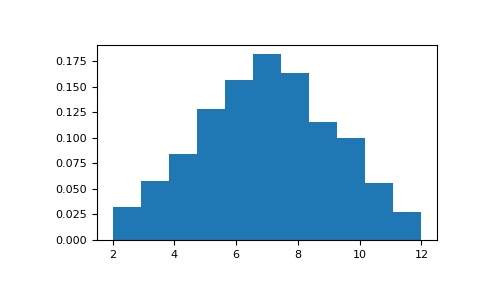#### Previous topic

numpy.random.RandomState.randint

#### Next topic

numpy.random.RandomState.random_sample

# numpy.random.RandomState.random_integers¶

method

`RandomState.``random_integers`(low, high=None, size=None)

Random integers of type np.int_ between low and high, inclusive.

Return random integers of type np.int_ from the “discrete uniform” distribution in the closed interval [low, high]. If high is None (the default), then results are from [1, low]. The np.int_ type translates to the C long integer type and its precision is platform dependent.

This function has been deprecated. Use randint instead.

Deprecated since version 1.11.0.

Parameters
lowint

Lowest (signed) integer to be drawn from the distribution (unless `high=None`, in which case this parameter is the highest such integer).

highint, optional

If provided, the largest (signed) integer to be drawn from the distribution (see above for behavior if `high=None`).

sizeint or tuple of ints, optional

Output shape. If the given shape is, e.g., `(m, n, k)`, then `m * n * k` samples are drawn. Default is None, in which case a single value is returned.

Returns
outint or ndarray of ints

size-shaped array of random integers from the appropriate distribution, or a single such random int if size not provided.

`randint`

Similar to `random_integers`, only for the half-open interval [low, high), and 0 is the lowest value if high is omitted.

Notes

To sample from N evenly spaced floating-point numbers between a and b, use:

```a + (b - a) * (np.random.random_integers(N) - 1) / (N - 1.)
```

Examples

```>>> np.random.random_integers(5)
4 # random
>>> type(np.random.random_integers(5))
<class 'numpy.int64'>
>>> np.random.random_integers(5, size=(3,2))
array([[5, 4], # random
[3, 3],
[4, 5]])
```

Choose five random numbers from the set of five evenly-spaced numbers between 0 and 2.5, inclusive (i.e., from the set):

```>>> 2.5 * (np.random.random_integers(5, size=(5,)) - 1) / 4.
array([ 0.625,  1.25 ,  0.625,  0.625,  2.5  ]) # random
```

Roll two six sided dice 1000 times and sum the results:

```>>> d1 = np.random.random_integers(1, 6, 1000)
>>> d2 = np.random.random_integers(1, 6, 1000)
>>> dsums = d1 + d2
```

Display results as a histogram:

```>>> import matplotlib.pyplot as plt
>>> count, bins, ignored = plt.hist(dsums, 11, density=True)
>>> plt.show()
```